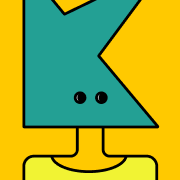Jeremy Kun
\$0+

190 ratings

# A Programmer's Introduction to Mathematics: Second Edition (pdf)

\$0+Jeremy Kun
190 ratings

A Programmer's Introduction to Mathematics uses your familiarity with ideas from programming and software to teach mathematics.

You'll learn about the central objects and theorems of mathematics, covering graphs, calculus, linear algebra, eigenvalues, optimization, and more. You'll also be immersed in the often unspoken cultural attitudes of mathematics, learning both how to read and write proofs while understanding why mathematics is the way it is. Between each technical chapter is an essay describing a different aspect of mathematical culture, and discussions of the insights and meta-insights that constitute mathematical intuition.

As you learn, we'll use new mathematical ideas to create wondrous programs, from cryptographic schemes to neural networks to hyperbolic tessellations. Each chapter also contains a set of exercises that have you actively explore mathematical topics on your own. By the end of the book, you will be able to learn mathematics on your own. In short, this book will teach you to engage with mathematics.

This is the ebook edition, a full-color pdf containing the complete contents of the book (the physical book is black and white). Due to the heavy use of mathematical typesetting, there is no plan for a ebook-reader-specific format (mobi, epub). This ebook is a simple pdf download.

\$

32.1 MB
398 pages

4.9
(190 ratings)
5 stars
96%
4 stars
3%
3 stars
1%
2 stars
0%
1 star
0%